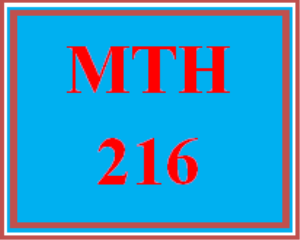# MTH 216T Wk 3 - Midterm Exam

PLDZ-13166 Free In Stock
\$ 0.00 USD
Description

***************************************************************

~~~~~~~~~~~~~~~~~~~~~~~~~~~~~~~~~~~~~~~~~~~~~~~~~

https://uopcourse.com/

***************************************************************

# Complete the midterm exam. You have one attempt at the exam. So, be sure you review all previous course materials before attempting the exam.

Question 1

Given a function described as equation y = 3x + 4, what is y when x is 1, 2, and 3?

7, 8, 9

3, 7, 11

3, 6, 9

7, 10, 13

Question 2

Given this equation for converting temperature from Celsius (c) to Fahrenheit (f): f = (9/5)c + 32

What is f when c is 30?

77

86

95

104

Question 3

What are m and b in the linear equation, using the common meanings of m and b?

1 + 4x + 6 - x = y

m is 3, b is 7

m is 4, b is 6

m is 7, b is 3

m is 6, b is 4

Question 4

The figure below shows costs for a current home mortgage versus a refinanced home mortgage. Refinancing has initial costs, but results in a lower monthly payment. Which best describes the lines?

The blue line is for refinancing, which starts cheaper but eventually is costlier

The blue line is for refinancing, which starts costlier but eventually is cheaper

The orange line is for refinancing, which starts cheaper but eventually is costlier

The orange line is for refinancing, which starts costlier but eventually is cheaper

Question 5

To draw a graph for y = 2x + 5, a person can draw a point at x of 0 and y of ___, a second point by going over 1 and up ___, and then draw a line through the points.

0, 5

2, 5

5, 2

2, 5

Question 6

A parking lot charges \$2 per hour for the first 4 hours, then \$3 per hour after that. Which equation(s) describes the total cost y as a function of the hours x?

y = 3x + 8

y = 2x + 12

y = 2x for x of 4 or less / y = 2x + 3x for x of 5 or more

y = 2x for x of 4 or less / y = 8 + 3(x - 4) for x of 5 or more

Question 7

Cells B1, C1, and D1 contain the values Seat1Row1, Seat1Row2, and Seat1Row3. If cells B1, C1, and D1 were selected, and autofill used to fill E1, F1, and G1, what would be the autofilled values?

Recent Reviews Write a Review
0 0 0 0 reviews